# Correlating Branch Prediction

Conditional Branches present in the programs significantly affect the performance of the system. So we need to come up with efficient branch prediction mechanism so as to get the branch target address with high accuracy and thus minimizing the stalls associated with control hazards.

In case if failure in correctly predicting target address, penalty will occur in terms of flushing the pipeline and bringing back the processors to a state that was there earlier when it was executing branch instruction.

Types of Branch Prediction Technique –
Branch prediction technique can be of two types:

1. Static Branch Prediction Technique
2. Dynamic Branch Prediction Technique

These are explained as following below.

1. Static Branch Prediction Technique :
In case of Static branch prediction technique underlying hardware assumes that either the branch is not taken always or the branch is taken always.
Let us understand branch prediction with an example code:

```//Code
int a=0;
while(a<5)
{
//branch instruction, condition either true or false
if(a%2==0)
{.....}
a++;
}```

Output –
Let us assume that underlying hardware has assumed that branch is not taken always. The output predicted by underlying hardware and actual output is shown in fig: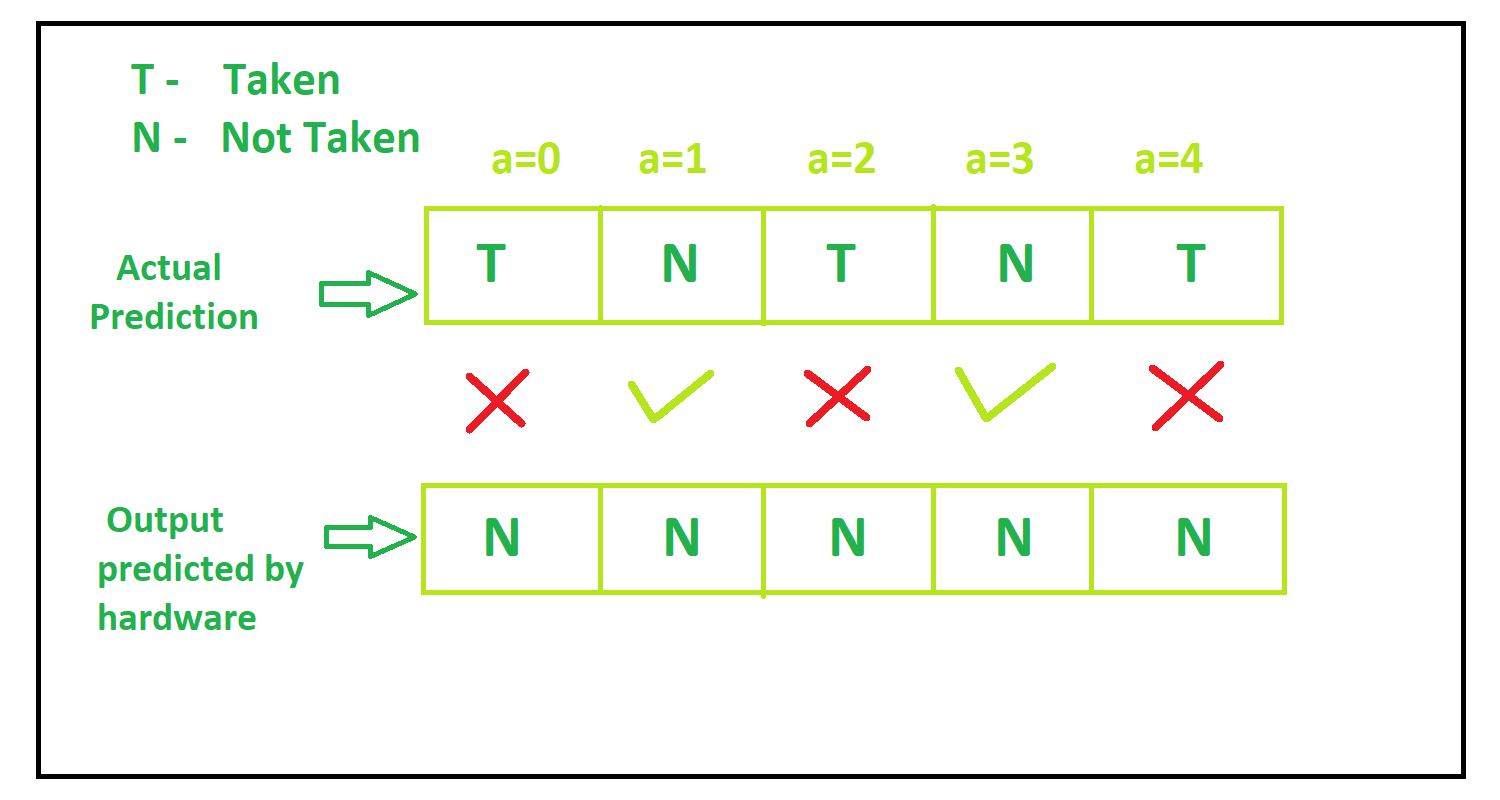2. Dynamic Branch Prediction Technique :
In Dynamic branch prediction technique prediction by underlying hardware is not fixed, rather it changes dynamically.This technique has high accuracy than static technique.

Some dynamic branch prediction techniques are:

1. 1-bit branch prediction technique
2. 2-bit branch prediction technique
3. Correlating branch prediction technique

These are explained as following below.

• 1-bit Branch Prediction Technique –
In this technique hardware changes its assumption just after one false assumption.For example if hardware assumes branch to be taken but actually branch is not taken, then in next step hardware assumes branch to be not taken and vice-versa.

1-bit branch prediction machine is shown in the fig below: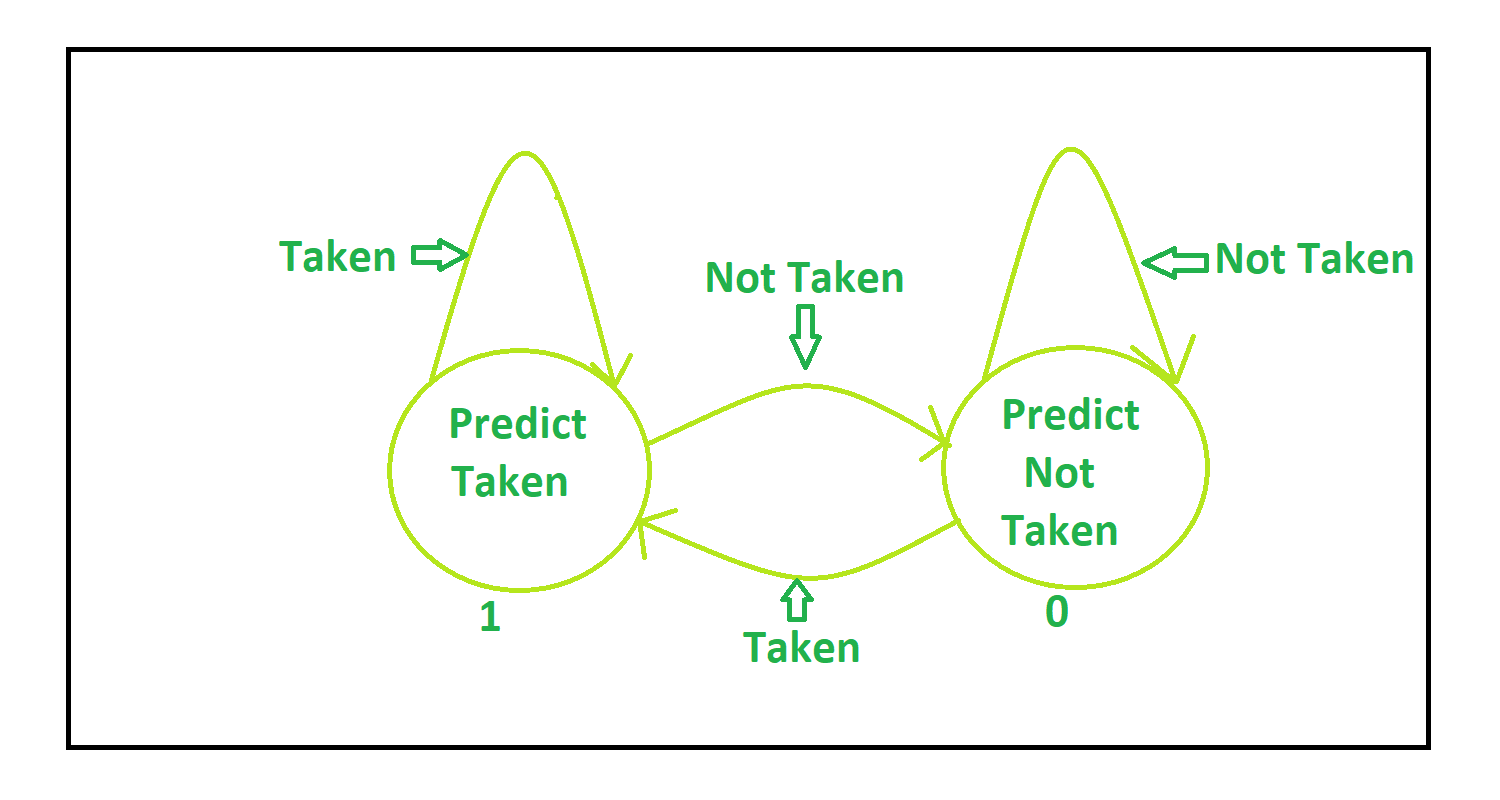Explanation –
At the beginning, lets say hardware assume branch to be take and so at a=0, branch is taken. At a=1, hardware assumes branch to be taken but branch is not taken.So now at a=2 hardware assumes branch not to be taken but branch is taken. At a=3 hardware assumes branch to be taken but branch is not taken. At a=4 hardware assumes branch not to be taken but branch is taken.

The output predicted by underlying hardware and actual output is shown in fig: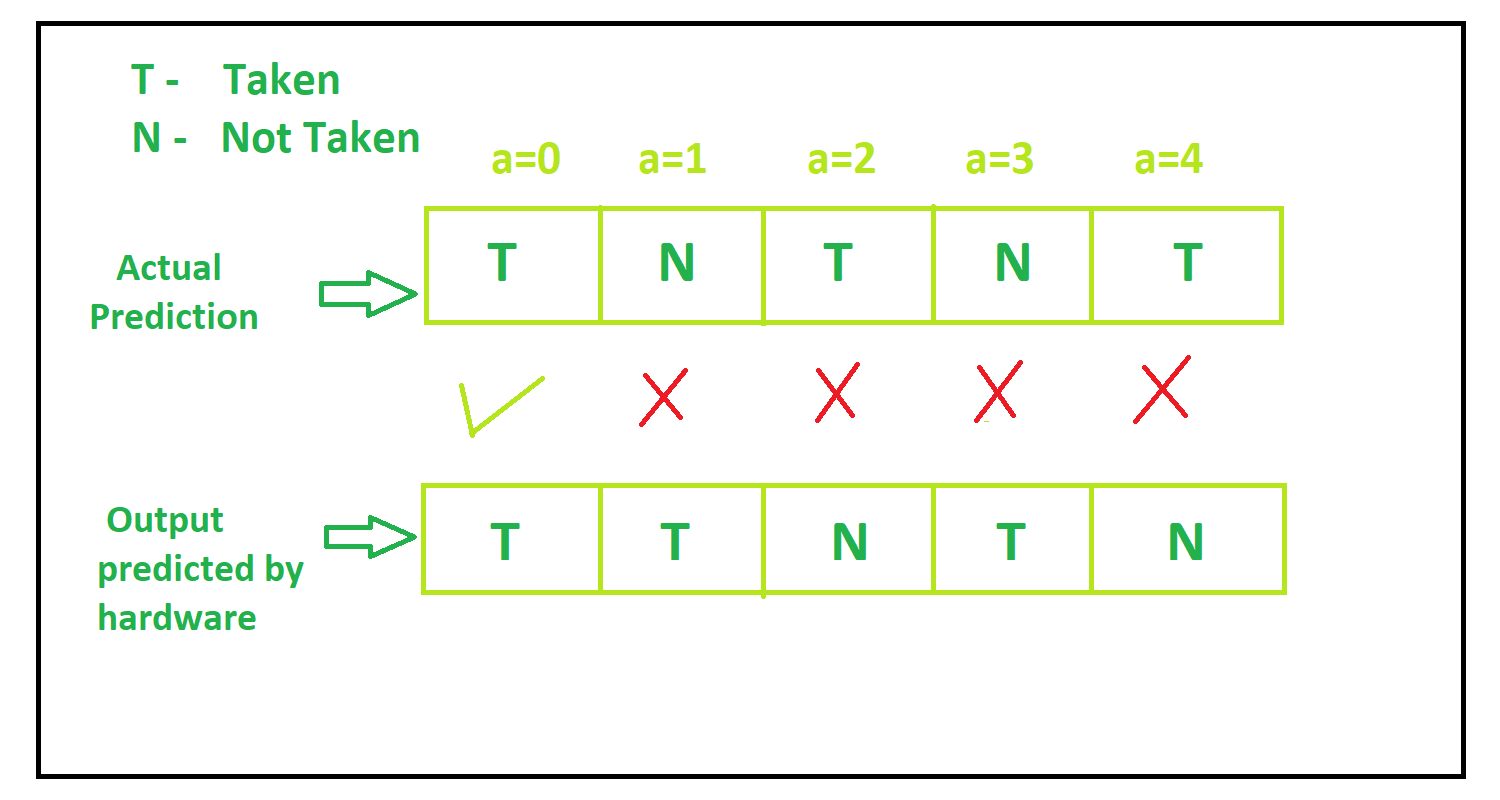• 2-bit Branch Predictor –
In this technique the underlying hardware does not changes its assumption just after one incorrect assumption, rather it changes its assumption after two consecutive wrong assumption and vice-versa.

2-bit branch prediction machine is shown in the figure: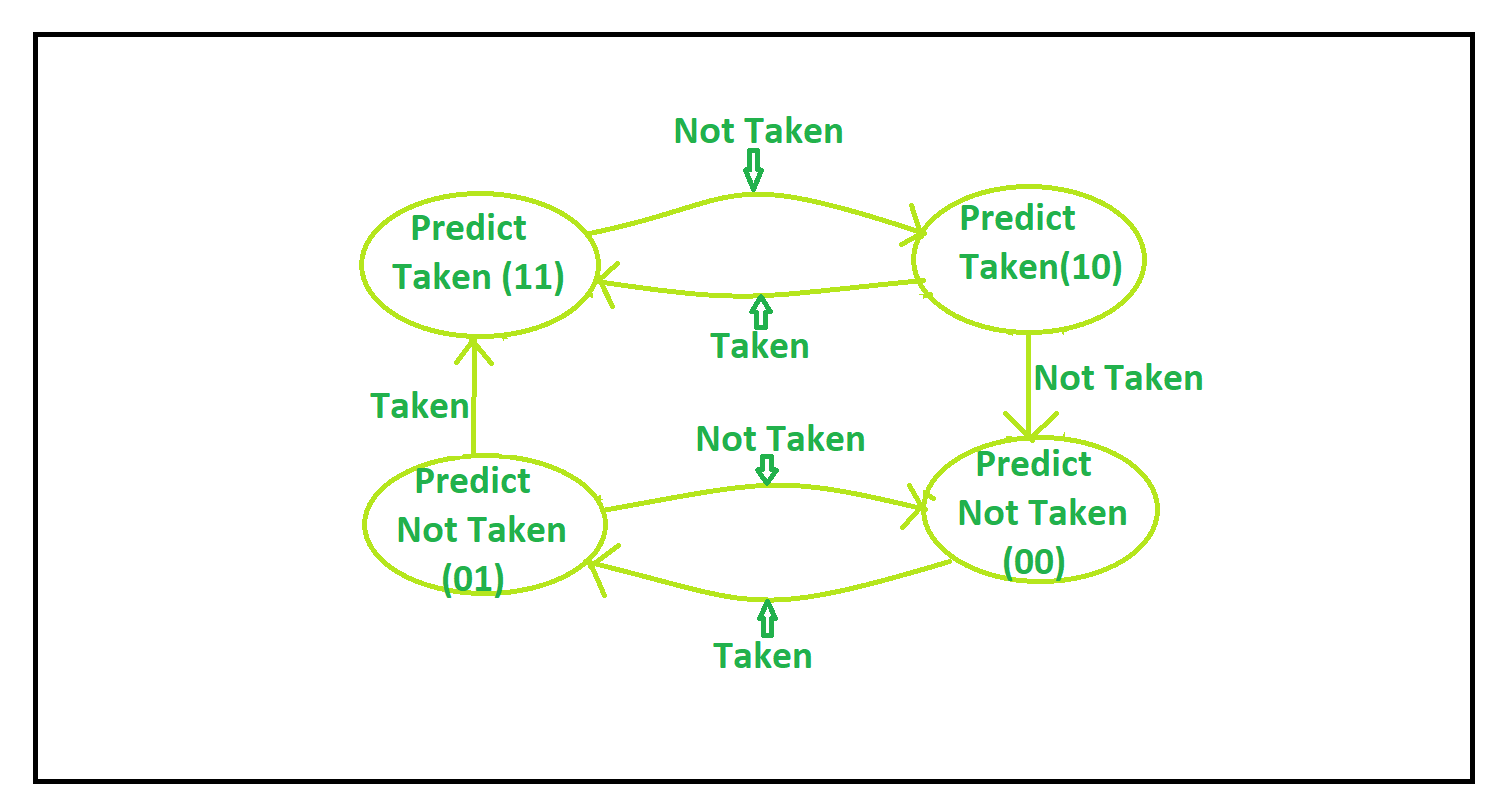Explanation –

1. Lets say when a=0 everything is reset(00) and so hardware assume branch not to be taken and branch is taken. So current state is (01)
2. When a=1, hardware assumes branch not to be taken and branch is not taken. So current state is (00)
3. When a=2, hardware assumes branch not to be taken and branch is taken.So current State is (01)
4. When a=3, hardware assumes branch not to be taken and branch is not taken. So current State is (10)
5. When a=4, hardware assumes branch not to be taken and branch is taken.So current State is(00)

The output predicted by underlying hardware and actual output is shown in fig: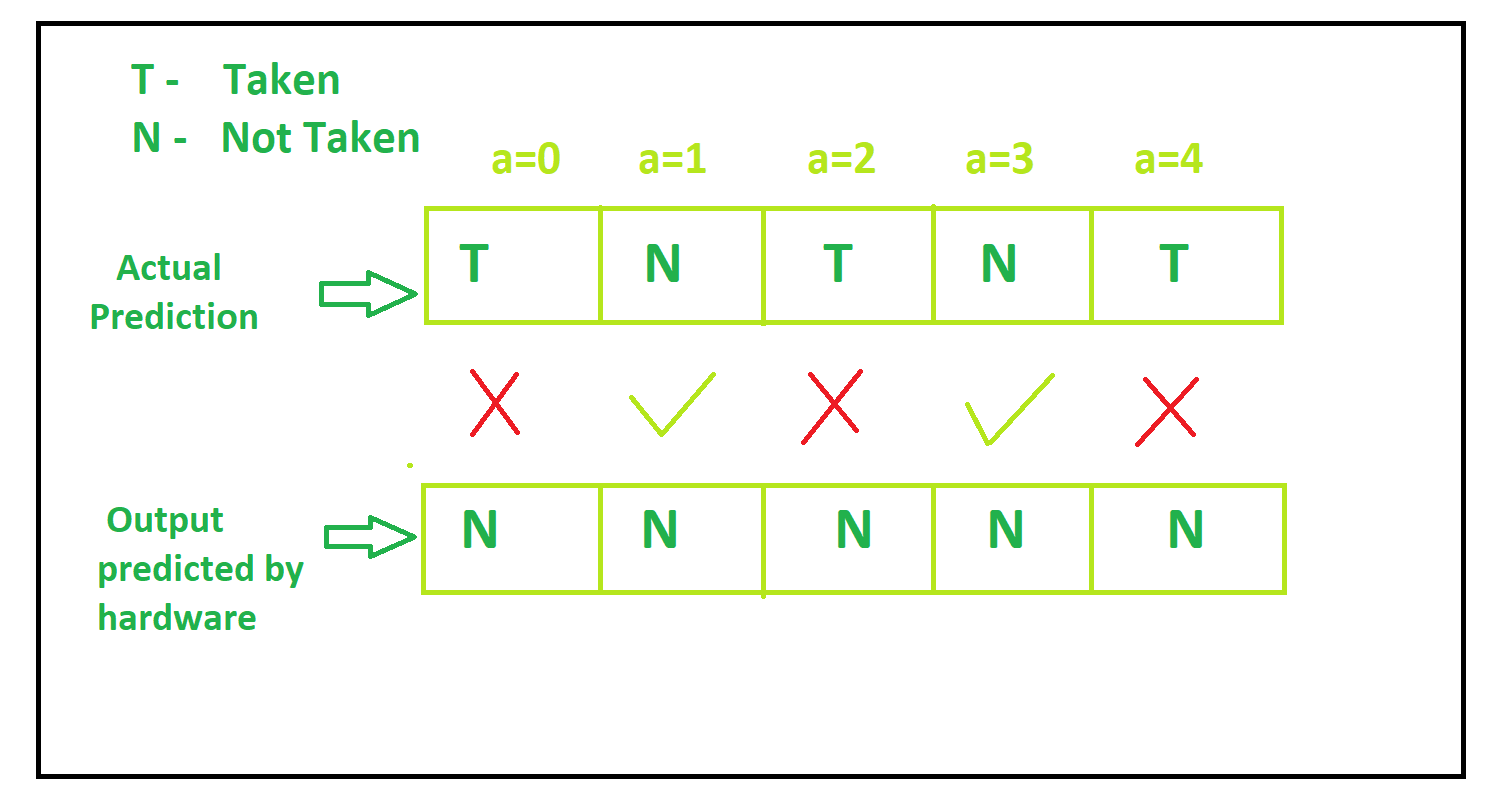• Correlating Branch Prediction –
We cannot get significant accuracy from 2-bit branch predictor also due to interference with other branches.So correlating branch prediction comes into picture which is also known as two-level branch predictor in which prediction accuracy is improved as it takes into consideration the recent behavior of other branches also.

Information Source –

• It uses k least significant bits of Branch Target Address which is fetched before.
• It also uses Local History Table(LHH) which is table of shift registers where shift register refers to the last outcome of m branches having same k least significant bits.
• It also uses Local Prediction Table to predict the outcome depending on the state in which it is present.

Example –
Lets illustrate and understand this prediction technique with the same example.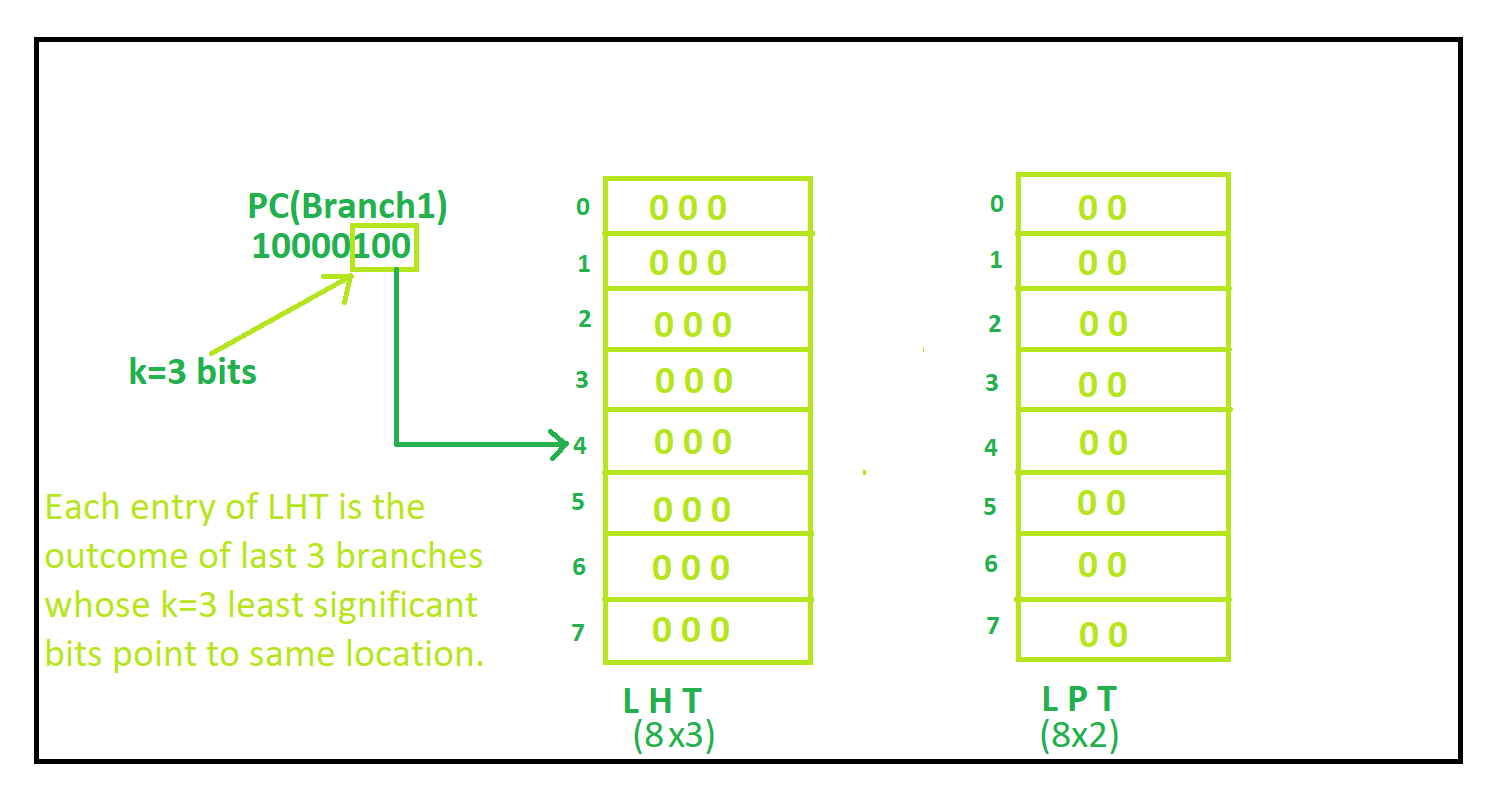Explanation Step By Step –
1. Least k(here k=3) significant bit is 100 i.e, 4 so this point to location 4 in Local History Table. At location 4, it contains 000 i.e, 0, so it points to location 0 in Local Prediction Table. It contains state 00 i.e branch not taken, but actually branch (0%2) is taken. So now both table gets updated.
Complete Process is shown in figure: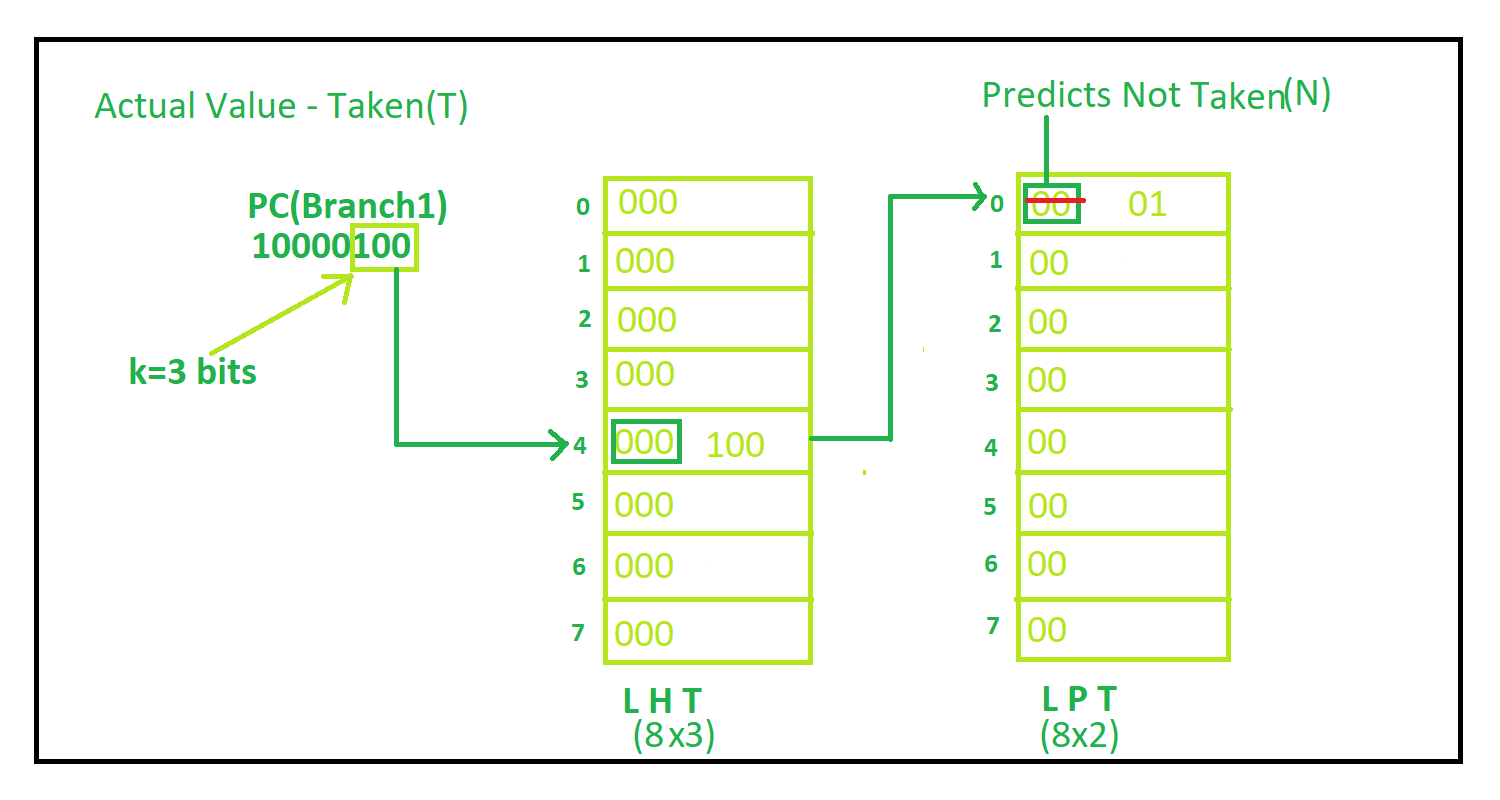2. At location 4, now it contains 100 in local history table, so it points to location 4 in Local Prediction Table. It contains state 00 i.e branch not taken, and actually branch is not taken. So accordingly table gets updated.
Complete Process is shown in figure: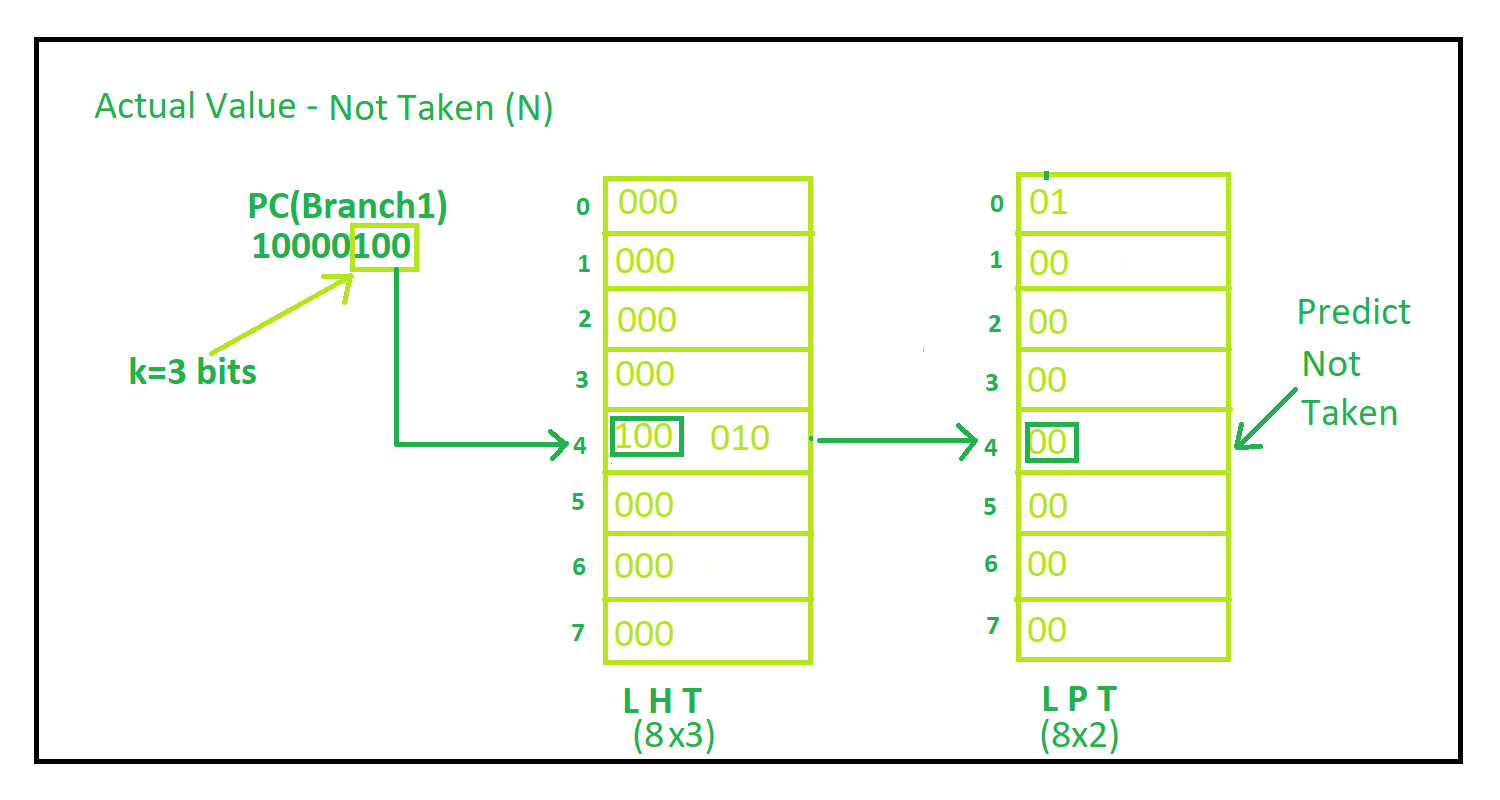3. t location 4 now it contains 010 in local history table, so it points to location 2 in Local Prediction Table. It contains state 00 i.e, branch not taken, and actually branch is taken. So accordingly table gets updated.
Complete Process is shown in figure: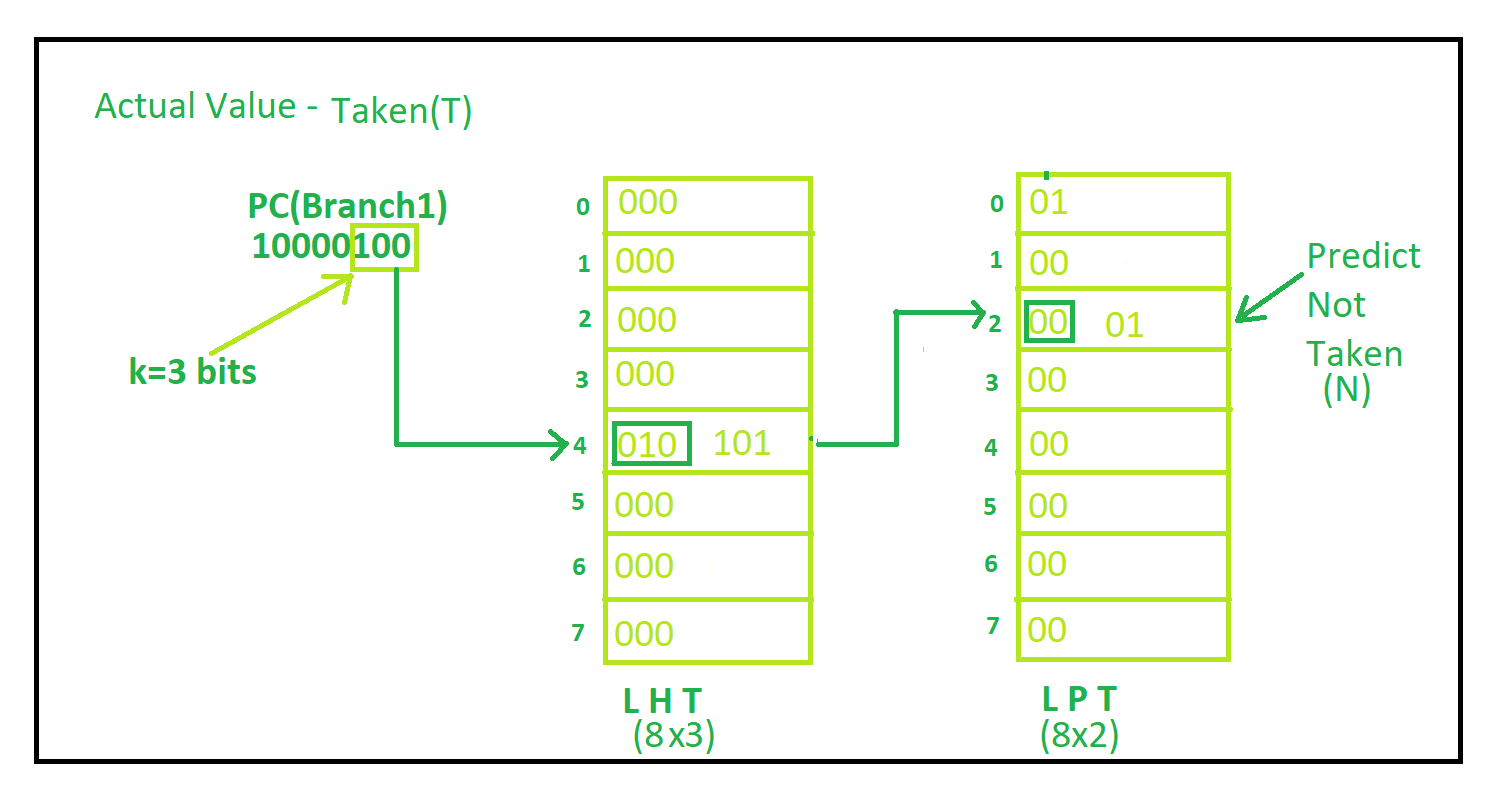4. At location 4, now it contains 101 in Local history table, so it points to location 5 in Local prediction table. It contains state 00 i.e, branch not taken, and actually branch is not taken. So accordingly table gets updated
Complete Process is shown in figure: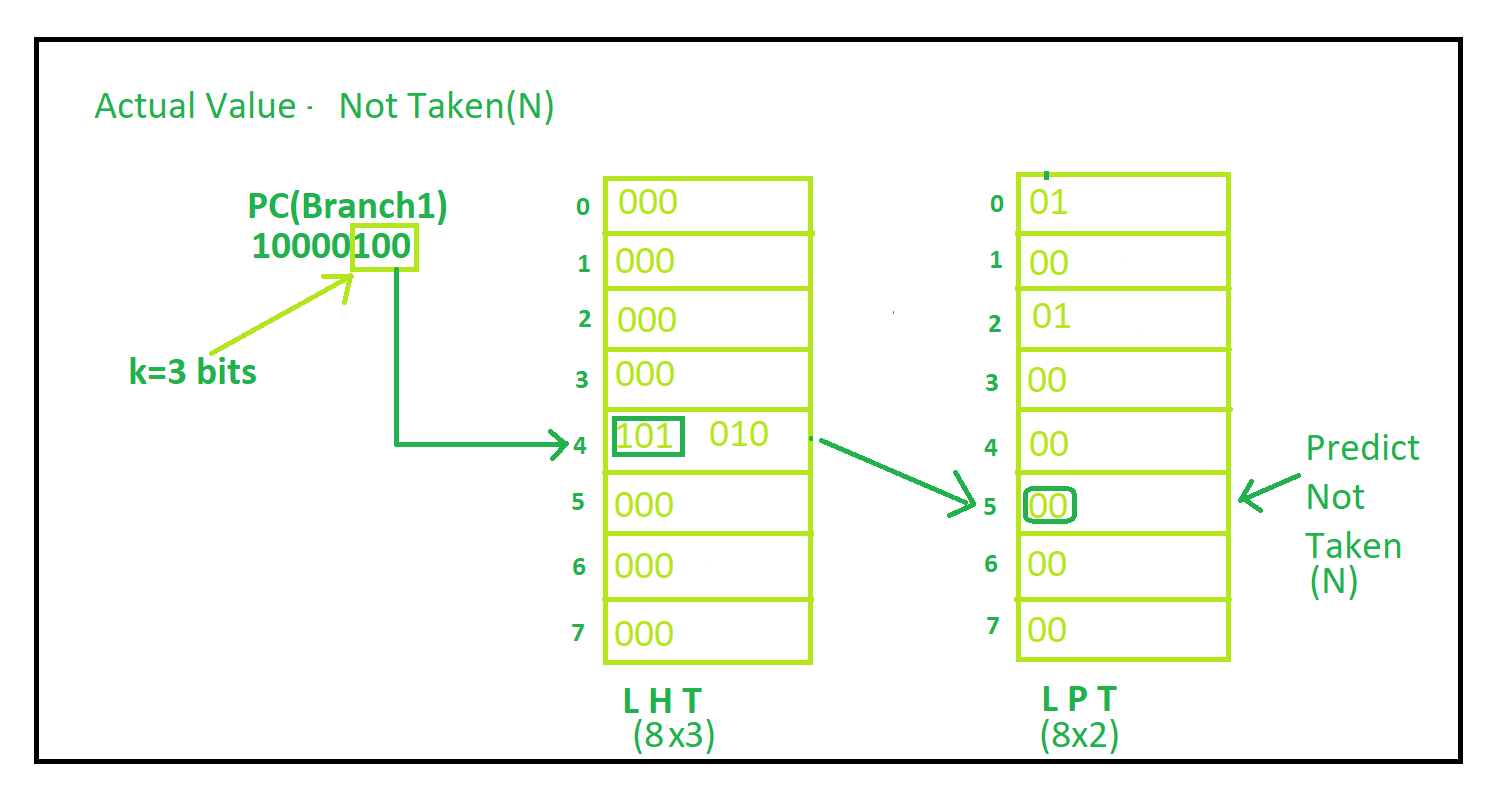5. At location 4 now it contains 010 in Local history table, so it points to location 2 in Local Prediction table. It contains state 01 i.e, branch not taken, and actually branch is taken. So accordingly table gets updated
Complete Process is shown in figure: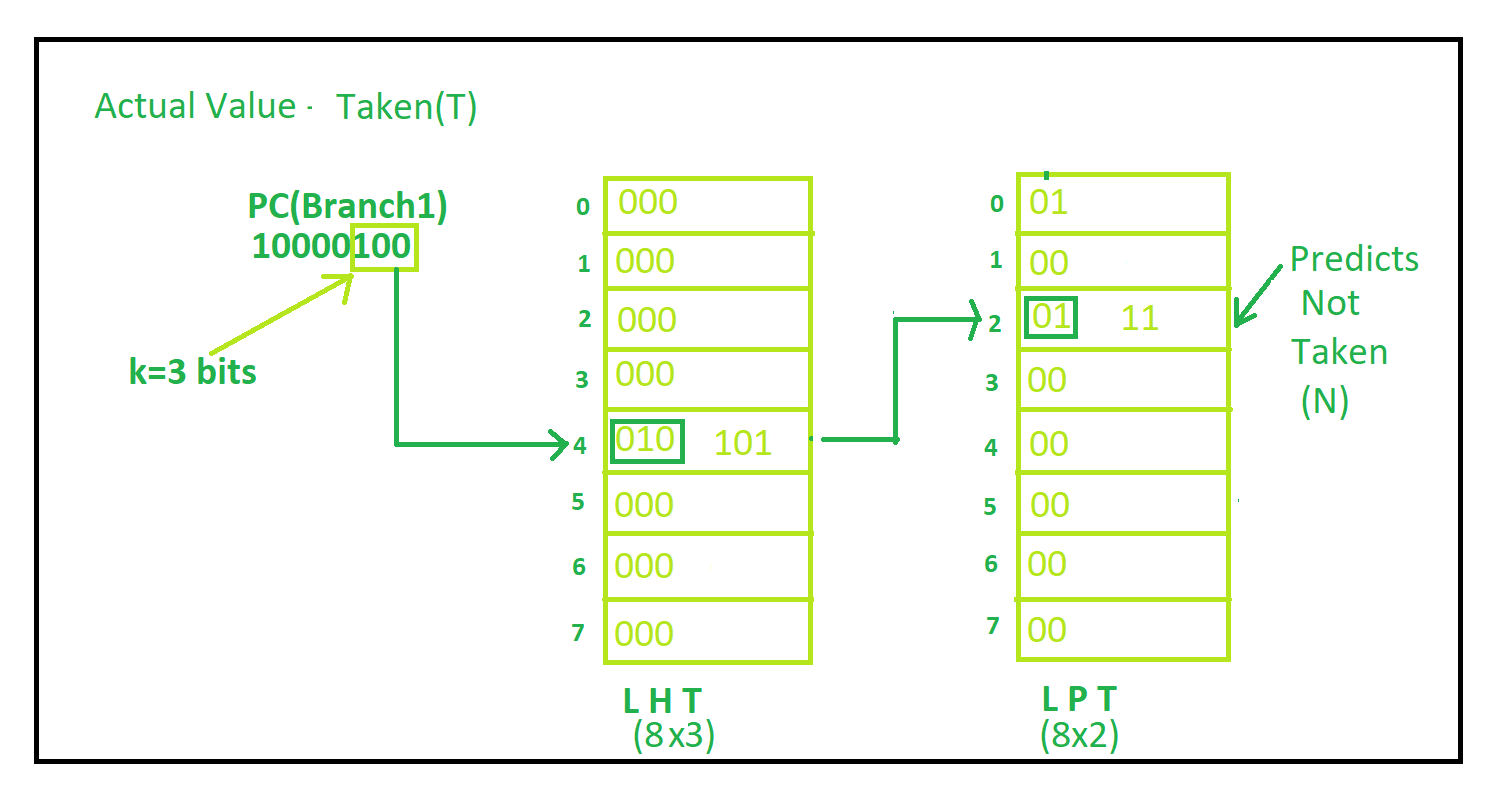The output predicted by underlying hardware and actual output is shown in fig: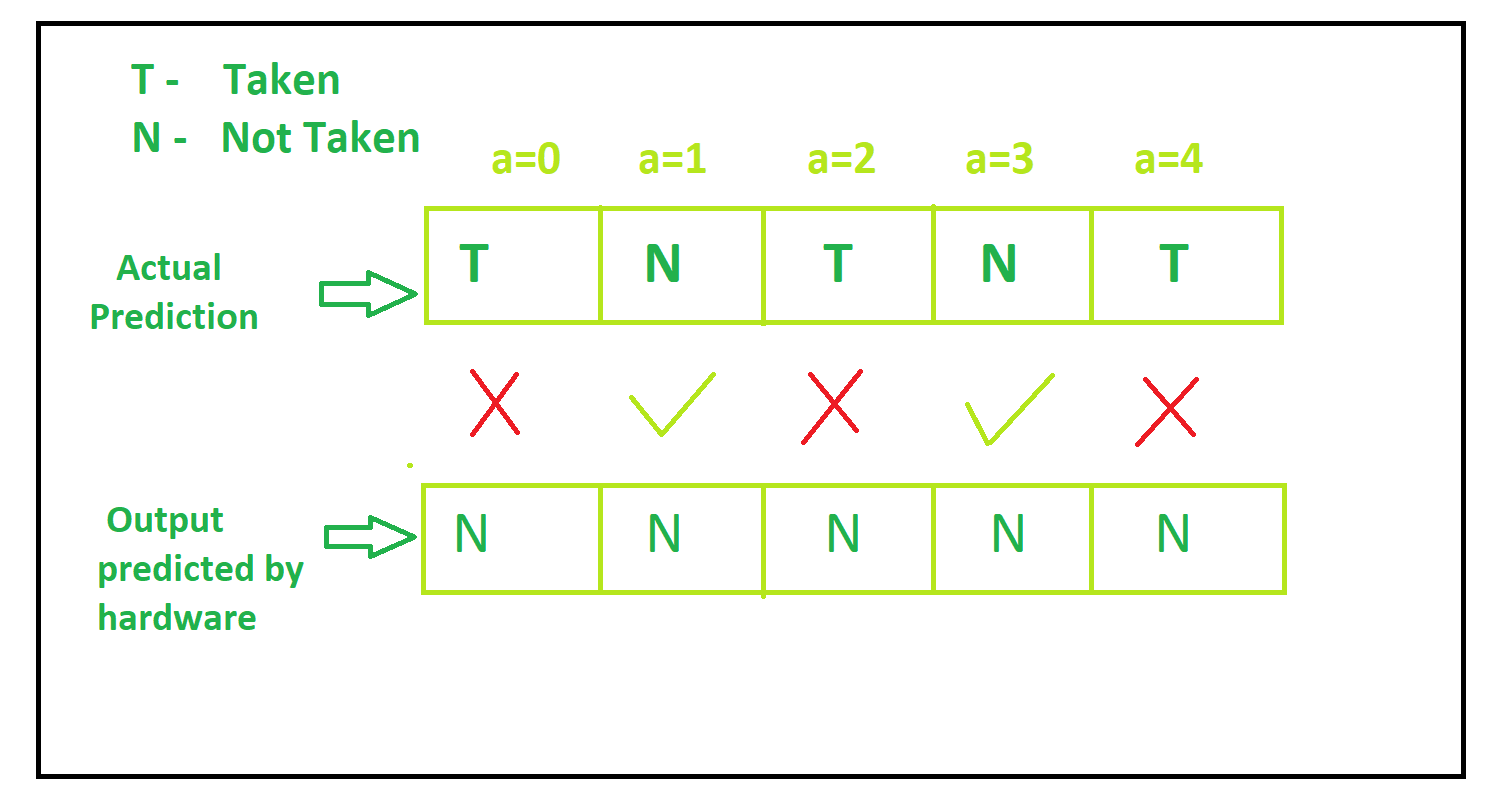So if there are more than one branches and process continues like this Correlating Branch Predictions gives highest accuracy above all technique.

Whether you're preparing for your first job interview or aiming to upskill in this ever-evolving tech landscape, GeeksforGeeks Courses are your key to success. We provide top-quality content at affordable prices, all geared towards accelerating your growth in a time-bound manner. Join the millions we've already empowered, and we're here to do the same for you. Don't miss out - check it out now!

Previous
Next